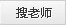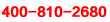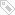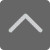• <th id="5kpb4"></th>
<tbody id="5kpb4"><noscript id="5kpb4"></noscript></tbody>
<th id="5kpb4"></th>

爱智康资讯站

资讯

北京

课程咨询: 4000-121-121

当前位置：北京学而思爱智康 > 小学教育 > 小学数学试题 > 正文北京小学四年级数学下册3单元检测题

2019-04-25 15:07:02 　来源：网络整理

北京小学四年级数学下册3单元检测题同学们人生没有后悔药，在我们还来得及的时候，好好努力学习，别将来走向社会后悔就晚了。下面就是小编为大家带来的北京小学四年级数学下册3单元检测题，希望可以帮助到大家。

想要了解【小学数学试题】的相关资料，请点击加入【爱智康小学交流福利群】，并直接向管理员“小康康”索取！爱智康小学交流福利群会不定期免费发放学习资料，小学政策等相关消息，请持续关注！

北京小学四年级数学下册3单元检测题

一、填空我最棒。(20分)

1. 填表

运算定律 加法交换律 加法结合律 乘法交换律 乘法结合律 乘法分配律

用字母表示

2. 在○里填上运算符号，在横线上填上合适的数。

(1)436-279-21=436-(279○_____)

(2)34×125×8=34×(___○____)

(3)120÷5÷4=120÷(___○____)

(4)49×38+15×38+38=(49+___+___)×___

3.在里填上“>”“<”或“=”。

651-45-355○651-(45+355)

12×15×25○12×(15+25)

80×125○10×8×125

15×(14+6)○15×14×6

二、大法官判对错。(对的画“√”，错的画“×”)(5分)

1.215-37+33=215-(67+33) ( )

2.240÷5÷4=240÷(5×4) ( )

3.102×47=100×47+2 ( )

4.85×16=85×10×6 ( )

5.99×125+125=(99+1)×125 ( )

三、择优录取。(将正确答案的序号填在括号里)(4分)

1.53×24+53×36=53×(24+36)运用了( )。

A.乘法交换律 B.乘法结合律

C.乘法分配律

2.125×4×25×8的简便算法是( )。

A.(125×8)×(4×25)

B.125×8+(4×25)

C.(125×25) ×(4×8)

3.792×99=792×100○792×1，○里应该填( )

A.+ B.- C. ×

4.三个数相乘，先乘前两个数或者先乘后两个数，积不变，这叫乘法( )律。

A.交换 B.结合 C.分配

四、数学门诊部。(对的画“√”，错的画“”，并改正)(3分)

1. 675-(275+43)

=675-275+43

=400+43

=443

2. 76×99+76

=76×(99+1)

=76×100

=7600

3. 47×99

=47×100-1

=4700-1

=4699

五、我是神算手。(14分)

1.直接写出得数。

15×11= 2400÷25= 180-79-21= 25×13×4=

200÷5÷4= 480÷3÷8= 125×3×8= 16×99=

2.计算下面各题，怎样简便就怎样算。

214×27-14×27 478-163-137 5000÷125÷8

281+93+119+207 99×34

六、下面的算式分别运用了哪些运算定律?(5分)

1.49+137=137+49

2.19×4=4×19

3.172+39+28+261=(172+28)+(39+261)

4.(8×79) ×125=(8×125) ×79

5.32×43+32×57=32×(43+57)

七、我会按要求做题。(先计算，再○里填上“<”“>”或“=”)(7分)

1. 490÷7+560÷7= (490+560) ÷7=

490÷7+560÷7○ (490+560) ÷7

2. 340÷2+480÷2= (340+480) ÷2=

340÷2+480÷2○(340+480) ÷2

从上面的两组算式中，你出现了什么规律?(用字母a、b、c)

八、列式计算。(15分)

1.125与79的积加上125与21的积，和是多少?

2.除数是32，商是15，余数是9，被除数是多少?

3.777与560的差，再除以7，商是多少?

九、解决生活中的问题。(27分)

一本漫画书有188页，林林第一天看了57页，第二天看了43页，还剩下多少页没看完?

参考答案：

一、1、

运算定律 加法交换律 加法结合律 乘法交换律 乘法结合律 乘法分配律

用字母表示 a+b=b+a (a+b)+c=a+(b+c) a×b=b×a (a×b)×c=a×(b×c) (a+b)×c=ab+ac

2、(1)+21 (2)(125×8) (3)5×4 (4) (49+15_+_1__)×38

3、= > = <

二、× √ × × √

三、1.C 2.A 3.B 4.B

四、× √ ×

五、1、165 96 80 1300 10 20 3000 1584

2、5400 178 5 700 3366 5700

六、1、加法交换律 2、乘法交换律、3、加法结合律

4、乘法结合律 5、乘法分配律

七、1、= 2、= 发现：a÷c+b÷c=(a+b)÷c

八、1、125×79+125×21=12500

2、32×15+19=499

3、(777-560)÷7=-31

九、188-57-43=88(页)

小编推荐：

以上就是小编特意为大家整理的北京小学四年级数学下册3单元检测题希望对有需要的同学提供帮助，大家如果在学习中有什么疑问，欢迎拨打爱智康免费电话：!那里有专业的老师为大家解答。

2019年精品学习包：

•小学语|数|英

历年试卷、北京30所校练习题、名著|重点校巡礼|等100讲视频、纸质书籍领取

•初中九大学科

历年真题、期末|期中|志愿填报|重点校巡礼|等100讲视频、纸质书籍领取

•高中九大学科

历年真题、期末|期中|志愿填报|重点校巡礼|等100讲视频、纸质书籍领取

•更新中

学习包内容持续更新中......标签：
你可能感兴趣的文章
热门推荐
课程推荐
小学知识点汇总• 全国课程在线咨询
• 咨询热线：4000-121-121
• 扫描注册有礼

• 让学习更有效广东快乐十分下载手机版2017_广东快乐十分下单平台 宅男| 克拉维茨出演猫女| duang| 感恩节| 台湾花莲海域地震| s9| 王一博| 唐伯虎点秋香| s9| 张天爱|